# Negative binomial distribution

Jump to: navigation, search

A probability distribution of a random variablewhich takes non-negative integer valuesin accordance with the formula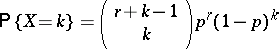(*)

for any real values of the parameters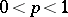and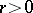. The generating function and the characteristic function of a negative binomial distribution are defined by the formulasand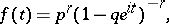respectively, where. The mathematical expectation and variance are equal, respectively, toand. The distribution function of a negative binomial distribution for the valuesis defined in terms of the values of the beta-distribution function at a pointby the following relation: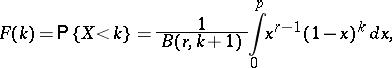whereis the beta-function.

The origin of the term "negative binomial distribution" is explained by the fact that this distribution is generated by a binomial with a negative exponent, i.e. the probabilities (*) are the coefficients of the expansion of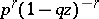in powers of.

Negative binomial distributions are encountered in many applications of probability theory. For an integer, the negative binomial distribution is interpreted as the distribution of the number of failures before the-th "success" in a scheme of Bernoulli trials with probability of "success"; in this context it is usually called a Pascal distribution and is a discrete analogue of the gamma-distribution. When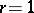, the negative binomial distribution coincides with the geometric distribution. The negative binomial distribution often appears in problems related to the randomization of the parameters of a distribution; for example, ifis a random variable having, conditionally on, a Poisson distribution with random parameter, which in turn has a gamma-distribution with density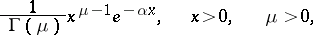then the marginal distribution ofwill be a negative binomial distribution with parameters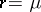and. The negative binomial distribution serves as a limiting form of a Pólya distribution.

The sum of independent random variableswhich have negative binomial distributions with parametersand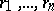, respectively, has a negative binomial distribution with parametersand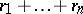. For largeand small, where, the negative binomial distribution is approximated by the Poisson distribution with parameter. Many properties of a negative binomial distribution are determined by the fact that it is a generalized Poisson distribution.